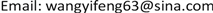1. 引言

2. 对牛顿第一运动定律的补充完善

3. 光速原理的推导

3.1. 基于物理角度推导光速原理

M U = M 1 + M 2 (2)

M U u U 2 = ( M 1 + M 2 ) u U 2 (3)

M U = M 1 + M 2 + M 3 + ⋅ ⋅ ⋅ (4)

M U u U 2 = ( M 1 + M 2 + M 3 + ⋅ ⋅ ⋅ ) u U 2 (5)

1) 在数值上，速度uU可以在静止( u U = 0 )和光速( u U = c )之间任意取值。从逻辑上说，如果认同物质是运动的观点，那么就没有不运动的质量体，也就是说没有静止的质量体，所以 u U = 0 的选项被排除；

2) 如果 u U < c ，从常识知道，一个质量体的总能量在数值上未必等于其质量和运动速度平方的乘积，换言之，当 u U ≠ c 时，宇宙总能量EU、宇宙总质量MU和运动速度uU三者之间的关系是不确定的；

3) 当 u U = c 时，与之相应的宇宙总能量EU

M U c 2 = E U (6)

3.2. 基于数学角度推导光速原理

1 = ∑ i = 1 ∞ a i , 0 < a i < 1 (7)

M U = M U ∑ i = 1 ∞ a i = ∑ i = 1 ∞ M U a i = ∑ i = 1 ∞ M i , 0 < M i < M U (8)

M U = M 1 + M 2 + M 3 + ⋅ ⋅ ⋅ (9)

M U u U 2 ≠ M 1 u 1 2 + M 2 u 2 2 + M 3 u 3 2 + ⋅ ⋅ ⋅ (10)

M U u 2 = M 1 u 2 + M 2 u 2 + M 3 u 2 + ⋅ ⋅ ⋅ = ( M 1 + M 2 + M 3 + ⋅ ⋅ ⋅ ) u 2 (11)

M U c 2 = ( M 1 + M 2 + M 3 + ⋅ ⋅ ⋅ ) c 2 = E U (12)

E U = M U c 2 ⇒ E U M U = c 2 ⇒ E U M U = c (13)

E i = M i c 2 (14)

E = M c 2 (15)

3.3. 光速原理与诺特定理之间的关系

20世纪初的数学家埃米·诺特提出了诺特定理，即对于力学体系的每一个连续的对称变换，都有一个守恒量与之对应。宇宙中的所有质量体构成一个最大的力学体系。根据诺特定理，宇宙的总能量和总动量肯定是守恒的，但是诺特定理并没有告诉我们如何计算，也没有指出守恒量具体为多少。光速原理给出了这些问题的答案。

E U c = c M U = P U (16)

PU的量纲为质量 × 速度，它是动量的量纲。在宇宙质量守恒、光速数值不变的前提下，式(16)表明了宇宙动量守恒。

4. 从光速原理导出的推论

4.1. 光速的起源

4.2. 超光速的不可能性

4.3. 瞬间传输的不可能性

“瞬间”意味着时间无穷小，因为速度等于长度除以时间，则相应的速度无穷大。如果超光速是可能的，那么不停地超光速下去，逻辑上讲就有可能让速度趋于无穷大。速度无穷大的传输可以瞬间完成，这就是所谓的“瞬间传输”。这里的前提是超光速；从逻辑上说，如果认可上述超光速的不可能性，那么该前提就是不成立的，随之相关的瞬间传输也是不可能的。

4.4. 在相同时间及相同方向上光子存在的唯一性

4.4.1. 光子的不可追赶性

4.4.2. 光子的辐射性

4.4.3. 光子运动方向的随机性

4.4.4. 光子空间分布的离散性

4.4.5. 光子空间分布的规律性

4.4.6. 质量体在接收外来光子的同时自身也在发射光子

4.4.7. 光子波动性观念的起源分析

5. 从向量分析的角度推导麦克斯韦方程组

A ⋅ C = A ⋅ C ⋅ cos θ ,     0 ≤ θ ≤ π (17)

A × C = u ⋅ A ⋅ C ⋅ sin θ ,     0 ≤ θ ≤ π (18)

∇ = i ∂ ∂ x + j ∂ ∂ y + k ∂ ∂ z (19)

∇ 算子与向量的点积称为该向量的散度  。例如， ∇ ⋅ A 称为向量A的散度， ∇ ⋅ C 称为向量C的散度。

∇ 算子与向量的叉积称为该向量的旋度  。例如， ∇ × A 称为向量A的旋度， ∇ × C 称为向量C的旋度。

5.1. 数学意义上的麦克斯韦方程组

E ⋅ H = 0 (20)

∇ ⋅ E = 0 (21)

∇ ⋅ H = 0 (22)

∇ × E = κ 1 ∂ H ∂ t (23)

∇ × H = κ 2 ∂ E ∂ t (24)

{ ∇ ⋅ E = 0 ∇ ⋅ H = 0 ∇ × E = κ 1 ∂ H ∂ t ∇ × H = κ 2 ∂ E ∂ t (25)

5.2. 物理意义上的麦克斯韦方程组

V m = J / C m = N ⋅ m / C m = N C

κ 1 ( 的 量 纲 ) ≡ 1 速 度 (27)

κ 2 ( 的 量 纲 ) ≡ 1 速 度 (28)

κ 1 × κ 2 ≡ 1 速 度 × 1 速 度 = 1 ( 速 度 ) 2 (29)

κ 1 = − 1 c ， κ 2 = 1 c (30)

{ ∇ ⋅ E = 0 ∇ ⋅ H = 0 ∇ × E = − 1 c ∂ H ∂ t ∇ × H = 1 c ∂ E ∂ t (31)

∇ 2 Ψ = 1 c 2 ∂ 2 Ψ ∂ t 2 (32)

∇ 2 = ∂ 2 ∂ x 2 + ∂ 2 ∂ y 2 + ∂ 2 ∂ z 2 (33)

κ 1 = − 1 ， κ 2 = μ 0 ε 0 (34)

κ 2 = μ 0 ε 0 = 1 c 2 ， c = 1 μ 0 ε 0 (35)

{ ∇ ⋅ E = 0 ∇ ⋅ H = 0 ∇ × E = − ∂ H ∂ t ∇ × H = 1 μ 0 ε 0 ∂ E ∂ t (36)

6. 麦克斯韦方程组描述光子辐射的不适用性

1) 因为距离相同，光速相同，所以必有 t 1 = t 2 ；

2) 光子q1没有电场/磁场转换，光子q2有电场/磁场转换， t 1 = t 2 必然意味着电场/磁场转换不需要时间，这与任何过程都需要时间的常识相矛盾；

3) 如果电场/磁场转换需要时间，则必然有 t 2 > t 1 ，这与1) 即 t 1 = t 2 相矛盾；

4) 如果光子的电场/磁场转换需要时间，但是这种转换瞬间完成，这意味着转换时间无穷小，但是仔细推敲一下就可以发现这种“瞬间”概念实际上隐含着一个前提条件即速度无穷大，这又与光速是一切物理速度的极限相矛盾；

5) 电场/磁场转换意味着能量分配，对于单个光子来说，牵涉到光子分裂问题。如果不加控制，光子分裂是随机性的；要做到具有类似波函数曲线那样的规律性，就需要某种机制来实现可控的光子分裂。目前来看，并没有这样一种机制；

6) 无数个光子的行为是单个光子行为的统计平均。如果单个光子不具有电场/磁场转换特性，无数个光子叠加起来具有电场/磁场转换特性，这在逻辑上和物理上均说不通。

7. 对若干基本概念的探讨7.1. 光子质量

E = h c λ = h v (37)

1   J = 1   kg ⋅ ( m / s ) 2 (38)

M = h λ c (39)

7.2. 光子的波长和频率是没有物理意义的辅助变量

7.3. 质量的种类有且只有一个

7.4. 时间不会对过程产生作用

8. 结束语